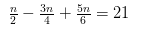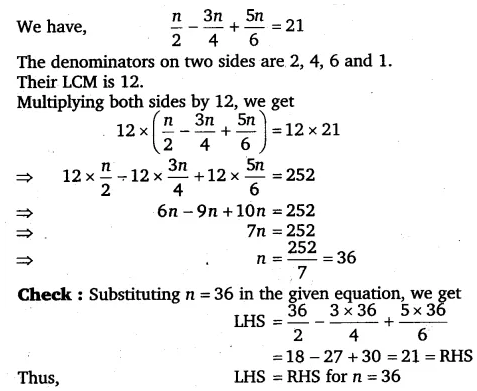# Solve the following linear equations: n/2 - 3n/4 + 5n/6 = 21

Solve the following linear equations: